Running BindingTutor

To plot a graph using BindingTutor, select the plotting options and binding model you would like to use, enter the parameters you want, and click the graph button. This section explains what plotting options and binding models are available.

Plotting Options

There are several sets of plotting options that can be selected. These options will apply to all graphed curves.

Experimental Method

There are three available experimental methods. Curves from different experimental methods cannot be plotted on the same graph, so changing the experimental method will automatically close an active graph.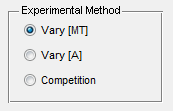Vary [L]: In this method, the concentration of P is held constant and the concentration of L is varied. The fraction of P bound is graphed on the y-axis. This is a "standard" binding experiment and simulates data from many standard experimental methods of measuring binding data, including SPR, fluorescence anisotropy, and tryptophan fluorescence, as well as cosedimentation assays.

Vary [P]: In this method, the concentration of L is held constant and the concentration of P is varied. The concentration of P bound is graphed on the y-axis. This kind of data cannot be collected using many standard binding assays such as SPR and fluorescence anisotropy, but it can be collected using a cosedimentation assay.

Competition: In this method, there are two L binding proteins, P and B. The concentration of P and the concentration of L is held constant while the concentration of B is varied. The fraction of P bound is plotted on the y-axis. This kind of data can be collected with many standard methods of measuring binding data, such as SPR, fluorescence anisotropy, tryptophan fluorescence, and cosedimentation assays.

Plotting Mode

BindingTutor can plot one curve at a time or it can plot two curves simultaneously and compare them. You may plot as many curves as you wish as long as you don't change the experimental method or X-axis settings. However, only two curves may be directly compared by the program.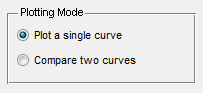X-axis

In Vary [L] mode, the X-axis can be set to either [L] total or [L] free.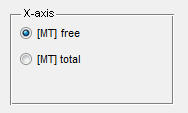[L] total is a known quantity in the experiment, while [L] free must be calculated. However, the familiar Langmuir Isotherm equation uses [L] free as its independent variable, and the quick method of determining K D by looking at the L concentration when the fraction of P bound is 0.5 only works if the x-axis is [L] free. In either X-axis mode the Y-axis will be the fraction of P bound.

In Vary [P] mode there are three possible kinds of plots. In the first two the Y-axis is the concentration of P bound to L, and the X-axis can be set to [P] free or [P] total. In addition, the data can be plotted in a Scatchard plot, where the X-axis is [P] bound and the Y-axis is is [P] bound/[P] free. This kind of plot is used to make the binding data linear. Binding data will be linear for simple interactions and a curved line in a Scatchard plot indicates the presence of cooperativity or other kinds of non-simple binding interactions.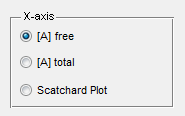Changing the x-axis mode will automatically close the active graph.

Number of Points

The number of points plotted may be specified.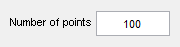The default number of points is 100. A larger number of points can be used if a smoother curve is desired. A smaller number of points will result in a faster calculation.

Binding Models

BindingTutor can plot curves for several binding models. For a detailed description of the math used to generate the curves, please see the Binding Model Mathematics chapter. Note that all models use the dissociation constant, KD.

The info button to the right of the model selection box provides a brief description of each model, reproduced below.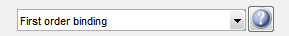First order binding: Simple P binds L interaction. This model is valid for any simple protein-protein or potein-ligand interaction.

Two binding sites: P can bind to two sites on each L. This model is valid for any protein-protein or protein-ligand interaction with two independent binding sites.

First Order Binding

First order binding simulates binding under standard first order conditions where one P interacts with one L, and all P-L interactions are identical.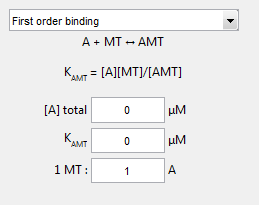For first order binding, you need to input the total concentration of P (or L) and the KD.. All of these must be positive numbers.

Two Binding Sites

In this model, P can bind to two sites per ligand, site 1 and site 2 with different dissociation constants.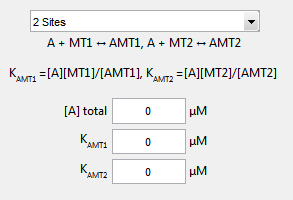In this model, you input the total amount of P (or L), the dissociation constant for P binding to L1sites, KD1and the dissociation constant for P binding to L2sites, KD2. All inputs must be positive numbers. Note that the total amount of L is the total amount of L1and L2.

This model cannot be graphed with an x-axis of [L] free.

Clone this wiki locally
You can’t perform that action at this time.